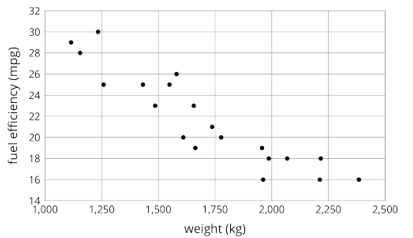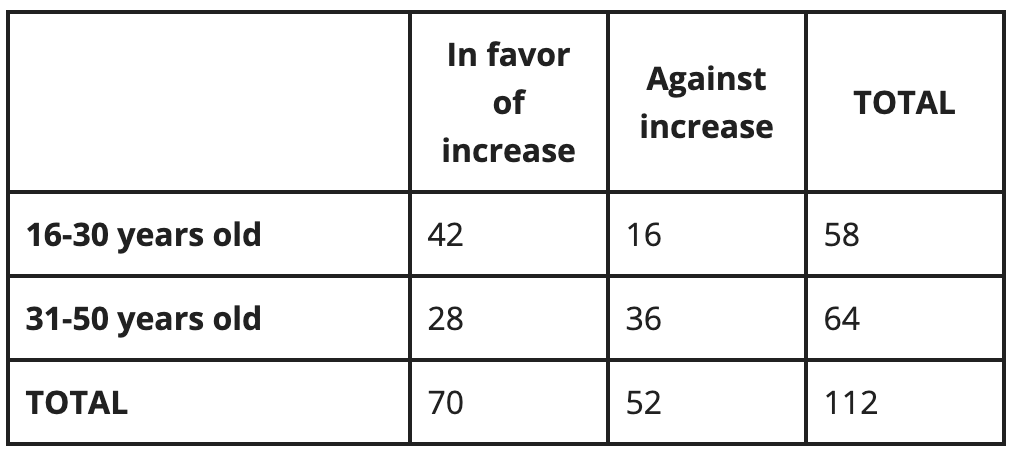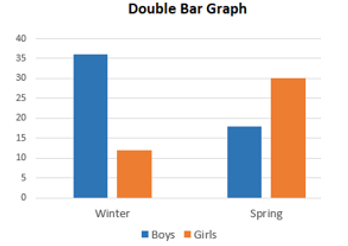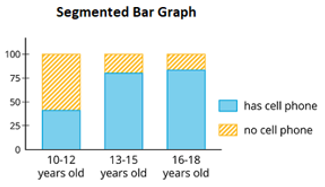# Bivariate Data

Students combine their knowledge of linear functions with knowledge of data representations and analysis to make the jump from univariate data in one variable to bivariate data in two variables.

## Unit Summary

In Unit 8, eighth-grade students make the jump from univariate data in one variable to bivariate data in two variables. They re-engage in the major work of the grade, analyzing scatterplots for positive or negative linear trends (MP.7), using lines to represent relationships between the variables, writing linear equations, interpreting these equations in context (MP.2), and using the equations to make predictions beyond the scope of the data (MP.4). Throughout the unit, students analyze scatterplots and two-way tables for trends in the data, asking themselves, Is there evidence in this graph or table to suggest an association between the variables? (MP.2)

Prior to eighth grade, students explored how and why data is collected—by thinking about statistical questions, samples, populations, and various ways to analyze data representations. Students worked with line plots, histograms, and box plots, and they considered what the shape, center, and spread of these data sets said about the data itself.

In high school, students’ understanding of statistics is formalized. They analyze bivariate data using functions, design and carry out experiments, and make predictions about outcomes based on probabilities. Students use their knowledge of association between variables as a basis for correlation. They develop nonlinear models for data and formally analyze how closely the model fits the data.

Pacing: 12 instructional days (9 lessons, 2 flex days, 1 assessment day)

For guidance on adjusting the pacing for the 2021-2022 school year, see our 8th Grade Scope and Sequence Recommended Adjustments.• Expanded Assessment Package
• Problem Sets for Each Lesson
• Student Handout Editor
• Vocabulary Package

## Assessment

This assessment accompanies Unit 8 and should be given on the suggested assessment day or after completing the unit.

## Unit Prep

### Intellectual Prep

?

#### Internalization of Standards via the Post-Unit Assessment

• Take the Post-Unit Assessment. Annotate for:
• Standards that each question aligns to
• Strategies and representations used in daily lessons
• Relationship to Essential Understandings of unit
• Lesson(s) that Assessment points to

#### Internalization of Trajectory of Unit

• Read and annotate the Unit Summary.
• Notice the progression of concepts through the unit using the Lesson Map.
• Essential Understandings
• Connection to Post-Unit Assessment questions
• Identify key opportunities to engage students in academic discourse. Read through our Teacher Tool on Academic Discourse and refer back to it throughout the unit.

#### Unit-Specific Intellectual Prep

 Model Example Scatter plotTwo-way tableDouble bar graphSegmented bar graph### Essential Understandings

?

• Patterns of association may exist between two variables defined in bivariate data. Looking at scatter plots of numerical data and two-way tables of categorical data can illuminate these associations.
• Relationships between variables in bivariate data can take several forms—positive or negative, linear or nonlinear, connected by relative frequency, etc.
• Knowing how two variables may be associated can inform predictions about data not in the data set.
• For data that is linearly related, a line is commonly used to represent the relationship between the variables.

### Vocabulary

?

segmented bar graph

double bar graph

outlier

cluster

scatter plot

bivariate data

positive/negative association

trend

linear/non-linear association

two-way table

line fit to data

To see all the vocabulary for this course, view our 8th Grade Vocabulary Glossary.

### Materials

?

• Calculators (1 per student)
• Graph Paper (1 sheet per student)
• Wikisticks or raw spaghetti (1 per student or small group)

# 1

8.SP.A.1

Define bivariate data. Analyze data in scatter plots.

# 2

8.SP.A.1

Create scatter plots for data sets and make observations about the data.

# 3

8.SP.A.1

Identify and describe associations in scatter plots including linear/nonlinear associations, positive/negative associations, clusters, and outliers.

# 4

8.SP.A.2

Informally fit a line to data. Judge the fit of the line and make predictions about the data based on the line.

# 5

8.SP.A.3

Write equations to represent lines fit to data and make predictions based on the line.

# 6

8.SP.A.3

Interpret the slope and $y$-intercept of a fitted line in context.

# 7

8.SP.A.4

Create and analyze two-way tables representing bivariate categorical data.

# 8

8.SP.A.4

Calculate relative frequencies in two-way tables to investigate associations in data.

# 9

8.SP.A.4

Complete two-way tables and identify associations in the data.

## Common Core Standards

Key: Major Cluster Supporting Cluster Additional Cluster

### Core Standards

?

##### Statistics and Probability
• 8.SP.A.1 — Construct and interpret scatter plots for bivariate measurement data to investigate patterns of association between two quantities. Describe patterns such as clustering, outliers, positive or negative association, linear association, and nonlinear association.

• 8.SP.A.2 — Know that straight lines are widely used to model relationships between two quantitative variables. For scatter plots that suggest a linear association, informally fit a straight line, and informally assess the model fit by judging the closeness of the data points to the line.

• 8.SP.A.3 — Use the equation of a linear model to solve problems in the context of bivariate measurement data, interpreting the slope and intercept. For example, in a linear model for a biology experiment, interpret a slope of 1.5 cm/hr as meaning that an additional hour of sunlight each day is associated with an additional 1.5 cm in mature plant height.

• 8.SP.A.4 — Understand that patterns of association can also be seen in bivariate categorical data by displaying frequencies and relative frequencies in a two-way table. Construct and interpret a two-way table summarizing data on two categorical variables collected from the same subjects. Use relative frequencies calculated for rows or columns to describe possible association between the two variables. For example, collect data from students in your class on whether or not they have a curfew on school nights and whether or not they have assigned chores at home. Is there evidence that those who have a curfew also tend to have chores?

?

• 8.F.A.3

• 8.F.B.4

• 8.F.B.5

• 6.RP.A.3.C

• 7.RP.A.3

• 6.SP.A.2

• 6.SP.B.4

• 7.SP.A.1

• 7.SP.A.2

• 7.SP.C.5

?

• S.ID.B.5

• S.ID.B.6

• S.ID.C.7

• S.ID.C.8

• S.ID.C.9

### Standards for Mathematical Practice

• CCSS.MATH.PRACTICE.MP1 — Make sense of problems and persevere in solving them.

• CCSS.MATH.PRACTICE.MP2 — Reason abstractly and quantitatively.

• CCSS.MATH.PRACTICE.MP3 — Construct viable arguments and critique the reasoning of others.

• CCSS.MATH.PRACTICE.MP4 — Model with mathematics.

• CCSS.MATH.PRACTICE.MP5 — Use appropriate tools strategically.

• CCSS.MATH.PRACTICE.MP6 — Attend to precision.

• CCSS.MATH.PRACTICE.MP7 — Look for and make use of structure.

• CCSS.MATH.PRACTICE.MP8 — Look for and express regularity in repeated reasoning.